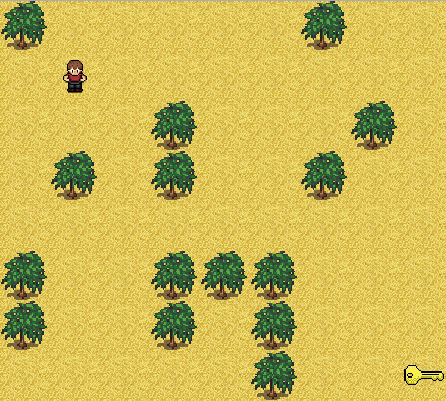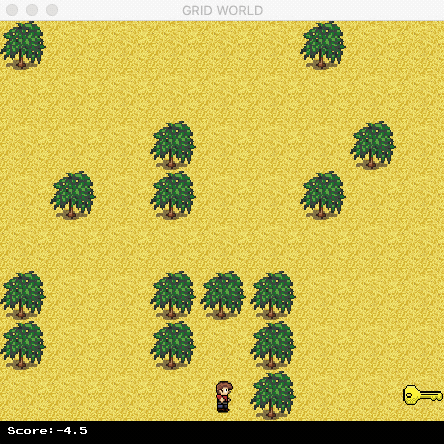## Grid World

In this sample code, we will explain how to create a graphical Grid World environment and integrate it into FruitAPI. Graphical environments are useful to examine deep RL methods with convolutional layers in the network.

In GridWorld, an agent starts off at one square (START) and moves (up, down, left, right) around a 2D rectangular grid of size (x, y) to find a designated square (END). The goal is to find the shortest path from START to END. To increase complexity, we assume that there are obstacles located in different squares of the world. The agent could not move into these dedicated locations, as shown in the following figure:#### BaseEngine

We will use Pygame to develop the game. Please install it before the tutorial.

It is quite straightforward to integrate the game into FruitAPI. We only need to implement the interface defined in `BaseEngine`, which is located in `/fruit/envs/games/engine.py`.

``````
class BaseEngine(object):
"""
Any game engine should follow this interface.
"""
def get_game_name(self):
"""
Returns name of the game
"""
pass

def clone(self):
"""
Clone itself
"""
pass

def get_num_of_objectives(self):
"""
The number of objectives, i.e., the number of reward signals in the game
"""
pass

def get_num_of_agents(self):
"""
The number of agents in the game
"""
pass

def reset(self):
"""
Reset the episode
"""
pass

def step(self, action):
"""
Ask agent to execute the specified ``action``
"""
pass

def render(self):
"""
Draw GUI
"""
pass

def get_state(self):
"""
Get current state (can be in graphical format)
"""
pass

def is_terminal(self):
"""
Is the episode terminated?
"""
pass

def get_state_space(self):
"""
Get the state space
"""
pass

def get_action_space(self):
"""
Get the action space
"""
pass

def get_num_of_actions(self):
"""
The number of possible actions that can be executed
"""
pass
```
```

#### GridWorld

Now, we create a class `GridWorld` that inherits `BaseEngine`. The constructor should include the following parameters:

• `render`: the environment is in render mode or not. We do not want to show the GUI while training but it is necessary while testing.
• `speed`: the number of frames per second.
• `max_frames`: it is quite often that the game may stuck forever if the agent cannot find the solution. Therefore, `max_frames` terminates the episode if the number of game frames exceed `max_frames`.
• `graphical_state`: a state of the environment can be an image or just a scalar value (the position of the agent).
• `seed`: a seed for random generator. Obstacles can be generated randomly or in fixed locations.
• `number_of_obstacles`: the number of obstacles in the game.
• `number_of_rows`: grid height size.
• `number_of_columns`: grid width size.
• `stage`: we can create predefined stages beforehand.
• `agent_start_x`: initial position of the agent in the grid (x axis).
• `agent_start_y`: initial position of the agent in the grid (y axis).

In the constructor, we use `seed` as follows:

``````if seed is None or seed < 0 or seed >= 9999:
self.seed = np.random.randint(0, 9999)
self.random_seed = True
else:
self.random_seed = False
self.seed = seed
np.random.seed(seed)``````
• The next step is to initialize the Pygame engine: `self.__init_pygame_engine()`
• Load a stage: `self.stage_map.load_map(self.current_stage)`
• Generate the agent: `self.__generate_players()`
• Finally, draw the first frame into the screen or into the buffer: `self.__render()`
• The full source code can be found in `/fruit/envs/games/grid_world/engine.py`

#### ResourceManager

This class is used to load (pre-load) all the resources (images, fonts) and manage them in an efficient way. The following resources are used in Grid World:

``````    LAND_TILE = "land4.png"
PLANT_TILE = "plant.png"
KEY_TILE = "key.png"
MINUS_TILE = 'minus.png'
GUY_L_1_TILE = "guy_l_1.png"
GUY_L_2_TILE = "guy_l_2.png"
GUY_R_1_TILE = "guy_r_1.png"
GUY_R_2_TILE = "guy_r_2.png"
GUY_U_1_TILE = "guy_u_1.png"
GUY_U_2_TILE = "guy_u_2.png"
GUY_D_1_TILE = "guy_d_1.png"
GUY_D_2_TILE = "guy_d_2.png"``````

The source code can be found here `/fruit/envs/games/grid_world/manager.py`

#### StageMap

`StageMap` is an efficent way to load a specific map in Grid World. We can define a map by the two following methods:

``````    def __build_map(self):

#########################################################################
#########################################################################
# STAGE 1
# Create a dynamic map

obs = random.sample(range(1, self.num_of_rows * self.num_of_columns - 1), self.num_of_obstacles)
self.map = [[-1 for _ in range(self.num_of_columns)] for _ in range(self.num_of_rows)]
self.map[-1][-1] = GlobalConstants.KEY_TILE
for o in obs:
r = int(o/self.num_of_columns)
c = int(o % self.num_of_rows)
self.map[r][c] = GlobalConstants.PLANT_TILE
#########################################################################

#########################################################################
# STAGE 2
# Create a static map
self.map = [[-1, -1,  1,  2, -1, -1, -1, -1, -1],
[-1,  0,  0,  0,  0,  0,  0,  0, -1],
[-1,  0, -1, -1, -1, -1, -1,  0, -1],
[-1,  0, -1, -1, -1, -1, -1,  0, -1],
[-1,  0,  0,  0, -1, -1, -1,  0, -1],
[-1, -1, -1, -1, -1, -1, -1,  0, -1],
[-1, -1,  0,  0,  0,  0,  0,  0, -1],
[-1, -1, -1, -1, -1, -1, -1, -1, -1]]
``````

#### Testing

Now we can test the game by using the following code. We use a random generator to let the agent moves randomly.

``````if __name__ == '__main__':
game = GridWorld(render=True, num_of_obstacles=15, graphical_state=False, stage=0,
number_of_rows=8, number_of_columns=9, speed=10, seed=100, agent_start_x=0, agent_start_y=0)
num_of_actions = game.get_num_of_actions()
game.reset()
state = game.get_state()

for i in range(10000):
random_action = np.random.randint(0, num_of_actions)
reward = game.step(random_action)
# next_state = Utils.process_state(game.get_state())
next_state = game.get_state()
is_terminal = game.is_terminal()
state = next_state

print('Action', random_action, 'Score Achieved', reward, 'Total Score', game.total_score, 'State', state)

if is_terminal:
print("Total Score", game.total_score)
game.reset()
break
``````

We can see that the random generator is impossible to find the solution. In the next post, we will use the Monte-Carlo method to train the agent to solve this problem.Contact us at `hello@fruitlab.org` or join our community at `https://www.facebook.com/groups/fruitlab/` to ask any questions.